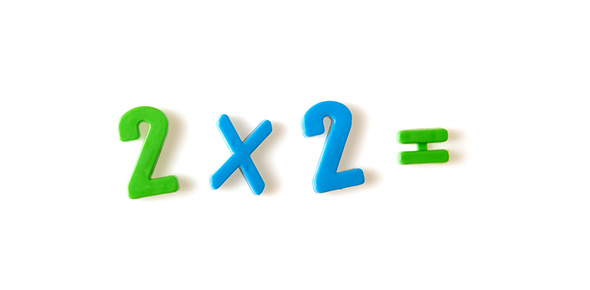# Shrr Multiplication 5

24 Questions | Total Attempts: 122SettingsThis is a timed multiplication quiz on the 5 time table.

Related Topics
• 1.
5 x 4 =
• 2.
5 x 0 =
• 3.
5 x 1 =
• 4.
5 x 2 =
• 5.
5 x 3 =
• 6.
5 x 5 =
• 7.
5 x 6 =
• 8.
5 x 7 =
• 9.
5 x 8 =
• 10.
5 x 9 =
• 11.
5 x 10 =
• 12.
5 x 11 =
• 13.
5 x 12 =
• 14.
1 x 5 =
• 15.
2 x 5 =
• 16.
3 x 5 =
• 17.
4 x 5 =
• 18.
6 x 5 =
• 19.
7 x 5 =
• 20.
8 x 5 =
• 21.
9 x 5 =
• 22.
10 x 5 =
• 23.
12 x 5 =
• 24.
11 x 5 =